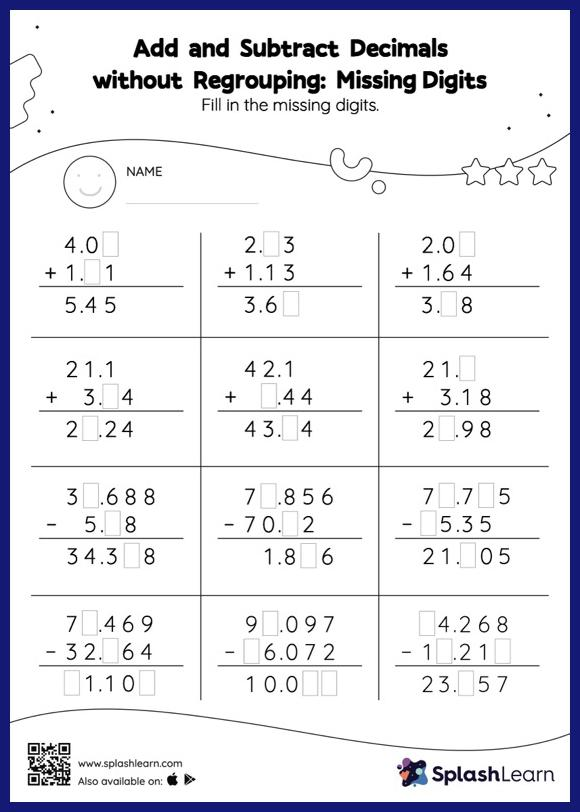# Add and Subtract Decimals without Regrouping: Missing Digits Worksheet

Home > Add and Subtract Decimals without Regrouping: Missing DigitsThis add and subtract decimals without regrouping worksheet consists of a set of questions on addition and subtraction, which help students develop fluency. Students find the missing number while adding and subtracting decimals by using the relationship between addition and subtraction. Add and subtract decimals without regrouping worksheet does not require them to regroup numbers.